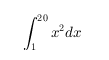# zlabel

## 语法

zlabel(txt)
zlabel(txt,Name,Value)
zlabel(ax,___)
h = zlabel(___)

## 说明

zlabel(txt) 使用文本 txt 为当前坐标区的 z 轴添加标签。重新发出 zlabel 命令可使新标签替换旧标签。

zlabel(txt,Name,Value) 还使用一个或多个 Name,Value 对组参数指定文本对象属性。
zlabel(ax,___) 将标签添加到 ax 指定的坐标区。此语法允许您指定要向其添加标签的坐标区。ax 可以位于先前语法中任何输入参数组合前面。
h = zlabel(___) 返回用作 z 轴标签的文本对象的句柄。该句柄对于以后修改标签非常有用。

## 示例

surf(peaks) zlabel('Height')figure surf(peaks) zlabel(123)MATLAB® 将 123 显示在 z 轴旁边。

figure surf(peaks) zlabel({'First Line';'Second Line'})figure surf(peaks) zlabel('Elevation','FontSize',12,... 'FontWeight','bold','Color','r')'FontSize',12 采用 12 磅字体显示标签文本。'FontWeight','bold' 将文本设置为粗体。'Color','r' 将文本颜色设置为红色。

tiledlayout(2,1) ax1 = nexttile; surf(ax1,peaks(30)) ax2 = nexttile; surf(ax2,peaks(45)) zlabel(ax2,'Height')z 轴添加标签并返回用作标签的文本对象。

surf(peaks) t = zlabel('Population Change');t.Color = 'red';## 输入参数

x = 42; txt = ['The value is ',num2str(x)];

• 使用字符串数组，其中每个元素包含一行文本，例如 ["first line","second line"]

• 使用元胞数组，其中每个元胞包含一行文本，例如 {'first line','second line'}

• 使用字符数组，其中每一行包含相同数量的字符，例如 ['abc'; 'ab ']

• 使用 sprintf 创建包含换行符的文本，例如 sprintf('first line \n second line')

• 如果将标签指定为分类数组，MATLAB® 将使用数组中的值，而不是类别。

• 词语 defaultfactoryremove 是保留字，作为标准字符引用时，标签中将不会出现。要在文本中单独显示这些单词中的任意单词，需在该单词之前放置反斜杠，例如 '\default''\remove'

### 名称-值对组参数

MATLAB 使用 FontWeight 属性从系统提供的字体中选择一种字体。并非所有字体都有加粗字体。因此，指定加粗字体仍可能得到普通字体。

• RGB 三元组是包含三个元素的行向量，其元素分别指定颜色中红、绿、蓝分量的强度。强度值必须位于 [0,1] 范围内，例如 [0.4 0.6 0.7]

• 十六进制颜色代码是字符向量或字符串标量，以井号 (#) 开头，后跟三个或六个十六进制数字，范围可以是 0F。这些值不区分大小写。因此，颜色代码 '#FF8800''#ff8800''#F80''#f80' 是等效的。

'red''r'[1 0 0]'#FF0000''green''g'[0 1 0]'#00FF00''blue''b'[0 0 1]'#0000FF''cyan' 'c'[0 1 1]'#00FFFF''magenta''m'[1 0 1]'#FF00FF''yellow''y'[1 1 0]'#FFFF00''black''k'[0 0 0]'#000000''white''w'[1 1 1]'#FFFFFF''none'不适用不适用不适用无颜色

RGB 三元组十六进制颜色代码外观
[0 0.4470 0.7410]'#0072BD'[0.8500 0.3250 0.0980]'#D95319'[0.9290 0.6940 0.1250]'#EDB120'[0.4940 0.1840 0.5560]'#7E2F8E'[0.4660 0.6740 0.1880]'#77AC30'[0.3010 0.7450 0.9330]'#4DBEEE'[0.6350 0.0780 0.1840]'#A2142F'• 'tex' - 使用 TeX 标记子集解释字符。

• 'latex' - 使用 LaTeX 标记解释字符。

• 'none' - 显示字面字符。

#### TeX 标记

^{ }上标'text^{superscript}'
_{ }下标'text_{subscript}'
\bf粗体'\bf text'
\it斜体'\it text'
\sl伪斜体（通常与斜体相同）'\sl text'
\rm常规字体'\rm text'
\fontname{specifier}字体名称 - 将 specifier 替换为字体系列的名称。您可以将此说明符与其他修饰符结合使用。'\fontname{Courier} text'
\fontsize{specifier}字体大小 - 将 specifier 替换为以磅为单位的数值标量值。'\fontsize{15} text'
\color{specifier}字体颜色 - 将 specifier 替换为以下颜色之一：redgreenyellowmagentablueblackwhitegraydarkGreenorangelightBlue'\color{magenta} text'
\color[rgb]{specifier}自定义字体颜色 - 将 specifier 替换为三元素 RGB 三元组。'\color[rgb]{0,0.5,0.5} text'

\alpha

α

\upsilon

υ

\sim

~

\angle

\phi\leq

\ast

*

\chi

χ

\infty

\beta

β

\psi

ψ

\clubsuit

\gamma

γ

\omega

ω

\diamondsuit

\delta

δ

\Gamma

Γ

\heartsuit

\epsilon

ϵ

\Delta

Δ

\spadesuit

\zeta

ζ

\Theta

Θ

\leftrightarrow

\eta

η

\Lambda

Λ

\leftarrow

\theta

θ

\Xi

Ξ

\Leftarrow

\vartheta

ϑ

\Pi

Π

\uparrow

\iota

ι

\Sigma

Σ

\rightarrow

\kappa

κ

\Upsilon

ϒ

\Rightarrow

\lambda

λ

\Phi

Φ

\downarrow

\mu

µ

\Psi

Ψ

\circ

º

\nu

ν

\Omega

Ω

\pm

±

\xi

ξ

\forall

\geq

\pi

π

\exists

\propto

\rho

ρ

\ni

\partial

\sigma

σ

\cong

\bullet

\varsigma

ς

\approx

\div

÷

\tau

τ

\Re

\neq

\equiv

\oplus

\aleph

\Im

\cup

\wp

\otimes

\subseteq

\oslash

\cap

\in

\supseteq

\supset

\lceil

\subset

\int

\cdot

·

\o

ο

\rfloor

\neg

¬

\nabla

\lfloor

\times

x

\ldots

...

\perp

\surd

\prime

´

\wedge

\varpi

ϖ

\0

\rceil

\rangle

\mid

|

\vee

\langle

\copyright

#### LaTeX 标记